JMSLTM Numerical Library 7.2.0
com.imsl.stat

## Class UserBasisRegression

• ```public class UserBasisRegression
extends Object```
Fits a linear function of the form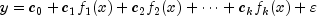, where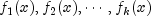are the user basis functions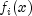evaluated at index values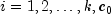is the intercept,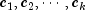are the coefficients associated with the basis functions, and is the random error associated with y. The coefficients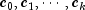are determined by least squares.

### Description

`UserBasisRegression` generalizes the concept of linear regression to user defined basis functions. The linear regression model is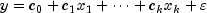, where are the k independent variables. `UserBasisRegression` generalizes this concept by setting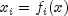, whereis any user defined function of.

This makes it easier for users to fit complex univariate models. For example, the `LinearRegression` class can be used to fit polynomials such as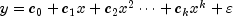, but this requires an input matrix where the ith column of that array contains the values of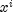.

With `UserBasisRegression`, these columns can be automatically generated. For this polynomial model, the user would define a user basis function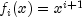. The `UserBasisRegression` class automatically inserts the necessary values into the regression equation and then calculates the coefficients and analysis of variance statistics.

Since the user provides a method for calculating the basis function, other more complex user basis functions are possible such as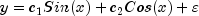. In this example, `nBasis`=2,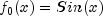, and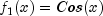.

Example 1, Example 2
• ### Constructor Summary

Constructors
Constructor and Description
```UserBasisRegression(RegressionBasis basis, int nBasis, boolean hasIntercept)```
Constructs a `UserBasisRegression` object
• ### Method Summary

Methods
Modifier and Type Method and Description
`ANOVA` `getANOVA()`
Get an analysis of variance table and related statistics.
`double[]` `getCoefficients()`
Returns the regression coefficients.
`void` ```update(double x, double y, double w)```
Adds a new observation and associated weight to the `RegressionBasis` object.
• ### Methods inherited from class java.lang.Object

`clone, equals, finalize, getClass, hashCode, notify, notifyAll, toString, wait, wait, wait`
• ### Constructor Detail

• #### UserBasisRegression

```public UserBasisRegression(RegressionBasis basis,
int nBasis,
boolean hasIntercept)```
Constructs a `UserBasisRegression` object
Parameters:
`basis` - a `RegressionBasis` basis function supplied by the user
`nBasis` - an `int` which specifies the number of basis functions
`hasIntercept` - a `boolean` which specifies whether or not the model has an intercept
• ### Method Detail

• #### getANOVA

`public ANOVA getANOVA()`
Get an analysis of variance table and related statistics.
Returns:
an `ANOVA` table and related statistics
• #### getCoefficients

`public double[] getCoefficients()`
Returns the regression coefficients.
Returns:
A `double` array containing the regression coefficients. If hasIntercept is false its length is equal to the number of variables. If hasIntercept is true then its length is the number of variables plus one and the 0-th entry is the value of the intercept.
Throws:
`SingularMatrixException` - is thrown when the regression matrix is singular.
• #### update

```public void update(double x,
double y,
double w)```
Adds a new observation and associated weight to the `RegressionBasis` object.
Parameters:
`x` - a `double` containing the independent (explanatory) variable.
`y` - a `double` containing the dependent (response) variable.
`w` - a `double` representing the weight
JMSLTM Numerical Library 7.2.0